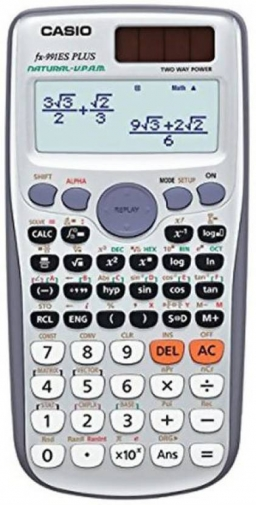# Three-quarters 29441

Peter bought a calculator. He saved three-quarters of its price himself, and half of the remaining amount was given to him by his grandfather. He still lacked 7 euros, which he borrowed from his brother. How much did the calculator cost?

x =  56 Eur

### Step-by-step explanation:Did you find an error or inaccuracy? Feel free to write us. Thank you!

Tips for related online calculators
Need help calculating sum, simplifying, or multiplying fractions? Try our fraction calculator.
Do you have a linear equation or system of equations and looking for its solution? Or do you have a quadratic equation?